# ggPMXAuthors: Amine Gassem, Irina Baltcheva, Christian Bartels, Thomas Dumortier, Souvik Bhattacharya, Inga Ludwig, Ines Paule, Didier Renard, Bruno Bieth

# Overview

ggPMX is an open-source R package freely available on CRAN since April 2019. It generates standard diagnostic plots for mixed effect models used in pharmacometric activities. The package builds on the R-package ggplot2 and aims at providing a workflow that is consistent, reproducible and efficient, resulting in high quality graphics ready-to-use in submission documents and publications. Intuitive functions and options allow for optimal figure customization and graphics stratification. ggPMX enables straightforward generation of PDF, Word or PNG output files that contain all diagnostic plots for keeping track of modeling results. The package is currently compatible with Monolix versions 2016 and later.

Using simple syntax, the toolbox produces various goodness-of-fit diagnostics such as: - residual- and empirical Bayes estimate (EBE)-based plots, - distribution plots, - prediction- and simulation-based diagnostics (visual predictive checks).

In addition, shrinkage and summary parameters tables can be also produced. By default, the PDF- or Word-format diagnostic report contains essential goodness-of-fit plots. However, these can be adapted to produce different sets of diagnostics as desired by the user, and any of the plots may be customized individually. The types of supported customizations include modifications of the graphical parameters, labels, and various stratifications by covariates.

# Installation

``````
# Either install from CRAN
install.packages("ggPMX")
# Or the development version from GitHub:
# install.packages("devtools")
devtools::install_github("ggPMXdevelopment/ggPMX")``````

# Testing the install

Once ggPMX is installed, you can test if everything is working well. If successful you should see a plot created after the following build-in example.

``````library(ggPMX)
ctr <- theophylline()
ctr %>% pmx_plot_eta_matrix()``````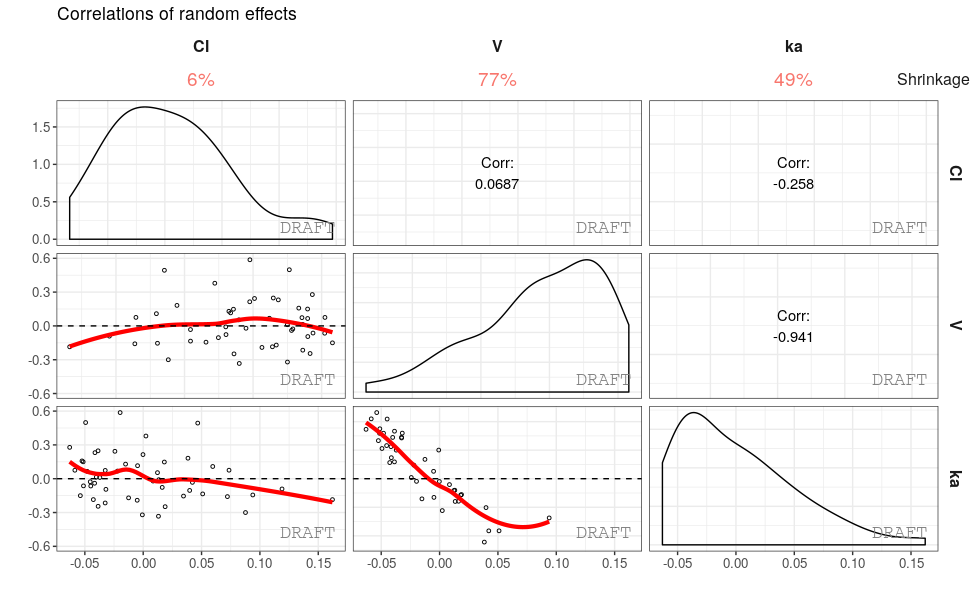# Cheatsheet# Feedback

ggPMX is now ready for inputs and enhancements by the pharmacometric community. - Please use package issues to fill in your feedback.

# Introduction

The `ggPMX` package generates standard diagnostic plots and tables for mixed effect models used in Pharmacometric (PMX) activities. The tool is built upon the ggplot2 package and is planned to support models developped either with Monolix or nlmixr software. The current release (0.9.9.9-7) supports models fitted with Monolix versions 2016 and later.

The package aims to provide a workflow that is consistent, efficient and which results in high quality graphics ready to use in official documents and reports. The package allows a high degree of flexibility and customization, yet providing an acceptable default setting. The package also allows to fully automate plots and report generation.

The general context is the analysis of mixed effect models fitted to data. ggPMX was developed in the framework of Pharmacometric activities, in which case the model is a population pharmacokinetic (PK) and/or pharmacodynamic (PD) model and the data is clinical or pre-clinical PK and/or PD data.

In the context of model building, evaluation and qualification, it is good practice to assess the goodness-of-fit of models by inspecting (qualitatively and quantitatively) a set of graphs that indicate how well the model describes the data. Several types of diagnostic plots allow to evaluate a mixed effects model fit, the most common being:

1. residual-based plots
2. prediction-based plots
3. empirical Bayes estimates (EBE)-based plots
4. simulation-based plots.

The following figures are examples of diagnotic plots.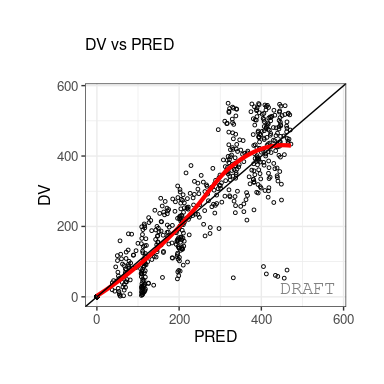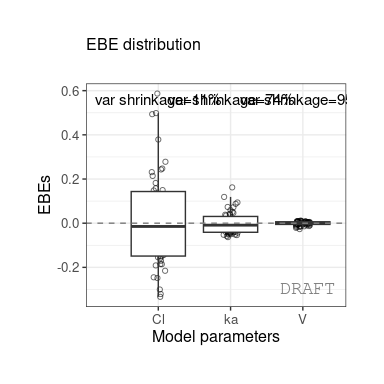This document introduces the ggPMX functionalities and syntax.

## Architecture

The high level architecture is represented in the figure below. The key components of the package are the following:

• Reader - reads model outputs from different sources (i.e.??text files containing population parameters, model predictions, individual random effects, simulations and data-related inputs like covariates) and restructures these outputs into standard formats for internal processing.
• Generator ??? processes outputs from Reader. It contains R language code to produce the plots and is factorized into a small set of flexible key functions. A set of default plots is defined in a configuration file. The configuration file can be adapted, e.g., to have different configurations for different types of modeling activities.
• Controller - serves as user interface. The user will call Generator functions via wrapper functions in the Controller to produce either all the default plots or selected plots of interest. In addition to editing the configuration, the user has different options to adapt aspects of the plots to specific requirements. Plots may be adapted by setting parameters of the wrapper functions that generate the plots; there exist additional wrapper functions to change aspects of the existing default plots. The plots are, in general, returned as ggplot objects that can be further customized using ggplot functionalities.
• Reporter - generates sets of graphs and tables and integrates them into an output file (Word or PDF) with annotations.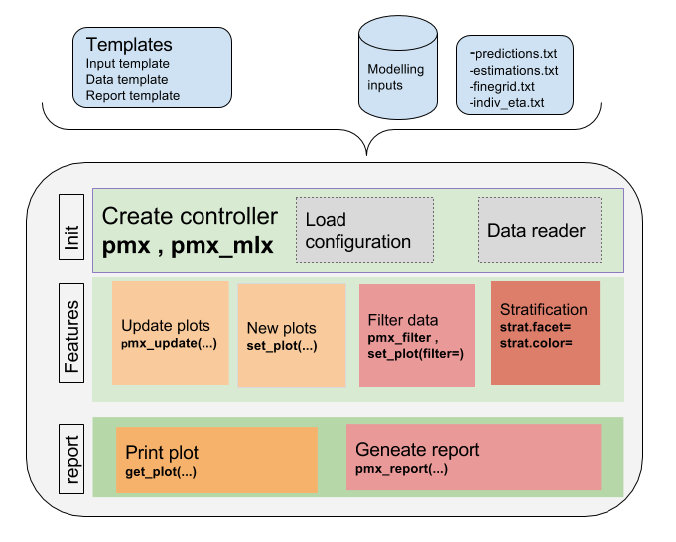The package is coded using object-oriented programming meaning that information is encoded in objects. The user can change or print the content of objets via functions. Such an implementation allows to have code that is modular and easily customizable.

## Workflow overview

The typical workflow of ggPMX is as follows:

1. The user creates the Controller using pre-defined configurations (yaml templates) for plot settings.
2. The Controller implicitly calls the Reader that reads and stores modelling outputs into a standard format. As a result, the Controller contains all available plots with their default values according to the configuration used.
3. The Generator allows to print the available plots by calling the corresponding functions. Plots can be modified by using optional arguments.
4. A call to the Reporter allows to create a pdf or docx report. The report Rmarkdown template can also be personalized.

The most important task for the user is the Controller creation. This step requires careful consideration because it involves different options according to the type of model (PK or PKPD) and software (Monolix or nlmixr) used for model fitting. The next section describes the Controller creation for the different possible cases.

Once the Controller is created, it implicitly calls the Reader and creates the diagnostic plots. The user can then generate the graphs by calling pre-defined functions as described in Section 3. The same syntax is used independent of the model structure (PK or PKPD model) and of the fitting software.

The Reporter creates one report per endpoint, i.e., one report for PK and one for each PD endpoint.

## Modeling datasets

For the sake of this document, three types of datasets are defined.

• The input modeling dataset is the one used for model fitting (the actual data). There are no particular requirements regarding this dataset.
• The output modeling datasets are those output from the fitting tool (Monolix or nlmixr). For version 1.0 of ggPMX, these datasets have to follow the structure of outputs of Monolix 2016 or 2018 (See Appendix for more details on Monolix requirements). A ???standard??? format for nlmixr output datasets will be defined for the the ggPMX version that allows usage of nlmixr as the fitting software.
• The ggPMX datasets are the ones created within (internal to) ggPMX. More details on the ggPMX datasets can be found in Section 2.4.3.

# Controller

A diagnostic session starts with the creation of a Controller. The Controller is the ???user interface??? of the package and allows to conrol all possible options. It is a container that stores configuration fields (model- and input data-related information), datasets and plots. It can be used as a reference object in which the user can see the names of the exisitng plots, the names of the `ggPMX` datasets, etc. The syntax of the Controller creation differs depending on the software used for model fitting and on the number of model endpoints (or outputs). This section presents different cases of Controller creation. For simplicity, the case of models with one single output is presented first (Section 2.1), then generalized to several outputs (Section 2.2). In Section 2.1 are presented other Controller creation functions that can be used with the different fitting softwares. Note that all these functions can also be used with models with several outputs. Section 2.7 provides details on the content of the Controller.

## Single-endpoint models

In general, models with only one endpoint (or output) are mostly PK models, but these could also be k-PD models.

To illustrate `ggPMX` functionalities, the single-endpoint built-in model called theophylline is used hereafter. The theophylline population PK example has the following characteristics:

• The input modeling data contains PK samples of 2 studies, each with 25 individuals who recieved a unique dose of 2000 mg theophylline.
• The model is a simple one-compartmental PK model with first-order absorption.
• The following covariates are used: weight (WT0) on volume (V) and clearance (Cl), age (AGE0), sex (SEX) and study (STUD) on clearance.
• Random effects on all three parameters (ka, Cl, V) are included.
• The residual error is proportional.

The input modeling dataset has the following columns:

``````#>   ID TIME  AMT   Y EVID WT0 AGE0 SEX STUD
#> 1  1  0.0 2000   0    1  87   73   1    1
#> 2  1  0.5    0 130    0  87   73   1    1
#> 3  1  1.0    0 228    0  87   73   1    1
#> 4  1  2.0    0 495    0  87   73   1    1
#> 5  1  3.0    0 484    0  87   73   1    1
#> 6  1  5.0    0 479    0  87   73   1    1``````

Note that the DVID (or CMT/YTYPE) column is missing, but since this is a single-endpoint model, it is not necessary in that case.

### Generic Controller creation with `pmx()`

The function `pmx()` is the generic function for greating a Controller. The user needs to specify a set of arguments such as the path to the model directory, the software used for model fitting (Monolix or nlmixr), the name of a configuration. A list of all existing configurations is provided in the Appendix. All mandatory arguments of `pmx()` are listed in Table .

The example below defines a Controller with the standing (standard) configuration.

``````theophylline_path <- file.path(system.file(package = "ggPMX"), "testdata", "theophylline")
work_dir          <- file.path(theophylline_path, "Monolix")
input_data_path   <- file.path(theophylline_path, "data_pk.csv")

ctr <- pmx(
sys       = "mlx",
config    = "standing",
directory = work_dir,
input     = input_data_path,
dv        = "Y",
dvid      = "DVID"
)``````

Note that the column ???DVID??? of data_pk.csv does not exist; however it is not needed here because there is only one single output of the model. As dvid is a mandatory argument, it still needs to be provided and was set arbritrarly to ???DVID??? in the example above.

The input dataset can be provided to ggPMX via its location (as in the example above) or as a data frame (maybe give an example). The modeling output datasets have to be in the location that is indicated as working directory (`work_dir` in the example above).

The above example of Contoller creation is wrapped in a function called ???theophylline()??? for quick reference:

``ctr <- theophylline()``

The following are optional arguments to the `pmx()` function (for details of each option, see the corresponding section):

• cats: character vector of categorical covariates (Section 2.3)
• conts: character vector of continuous covariates (Section 2.3)
• occ: character occasinal covariate variable name
• strats: character extra stratification variables (Section 2.3)
• settings: global pmxSettingsClass (`pmx_settings()`) shared between all plots
• endpoint: pmxEndpointClass (`pmx_endpoint()`) or integer or charcater of the endpoint code (Section 2.2)
• sim: pmxSimClass object for VPC generation.

### Models fitted with Monolix (versions 2016 and later)

####`pmx_mlx()`

The controller initialization can be simplified by using the Monolix controller `pmx_mlx()`, which is a wrapper function for `pmx()` with `sys="mlx"`. Note that the `sys` argument is no longer required.

``````ctr <- pmx_mlx(
config    = "standing",
directory = work_dir,
input     = input_data_path,
dv        = "Y",
dvid      = "DVID"
)``````

#### `pmx_mlxtran()`

The controller initialization can be simplified even further by using the Monolix controller `pmx_mlxtran()`. This function parses the mlxtran file of a Monolix project and assigns automatically the different fields necessary to the Controller creation. The only mandatory argument is file_name, the path to the mlxtran file.

``````mlxtran_path <- file.path(system.file(package = "ggPMX"),
"testdata", "1_popPK_model", "project.mlxtran")

ctr <- pmx_mlxtran(file_name = mlxtran_path)``````

The user can verify the content of the Controller and how parameters are assigned by printing it (see Section 2.4).

## Multiple-endpoint models

Models with more than one endpoint (or output) are mostly PKPD models, but these could also be, for example, PK binding models in which there are measurements and predictions of both PK and its target.

ggPMX produces one diagnostics report per endpoint. As a consequence, the endpoint (if more than one) should be set at the time of the Controller creation in order to filter the observations dataset and to keep only the values corresponding to the endpoint of interest. To handle this, the user creates an ???endpoint??? object using the function `pmx_endpoint()` having the following attributes:

• code (charcater): how the endpoint is coded in the input (modeling) dataset
• label: can be used in the title of some plots or for the report file name
• unit: used in the axis label for some plots
• files (list): `list(predictions="predictions1",finegrid ="finegrid1")`
• trans: whether the value must be transformed before being displayed and which transformation to use.

To illustrate the Controller creation with multiple-endpoint models, a built-in PKPD example is used. The input dataset is called pk_pd.csv and has the following columns.

``````#>    id time amt  dv dvid   wt sex age
#> 1 100  0.0 100   .    3 66.7   1  50
#> 2 100  0.5   .   0    3 66.7   1  50
#> 3 100  1.0   . 1.9    3 66.7   1  50
#> 4 100  2.0   . 3.3    3 66.7   1  50
#> 5 100  3.0   . 6.6    3 66.7   1  50
#> 6 100  6.0   . 9.1    3 66.7   1  50``````

The dvid column contains values=3 for PK (first endpoint) and dose and =4 for PD (second endpoint). Monolix2016 outputs are found in folder RESULTS/ which contains predictions1.txt and finegrid1.txt for PK predictions, and predictions2.txt and finegrid2.txt for PD predictions. The Endpoint and Controller objects are created as follows:

``````pkpd_path       <- file.path(system.file(package = "ggPMX"), "testdata", "pk_pd")
pkpd_work_dir   <- file.path(pkpd_path, "RESULTS")
pkpd_input_file <- file.path(pkpd_path, "pk_pd.csv")

ep <- pmx_endpoint(
code  = "4",
label = "some_label",
unit  = "some_unit",
file.code = "2", # will use predictions2.txt and finegrig2.txt
trans = "log10"
)

ctr <- pmx_mlx(
config    = "standing",
directory = pkpd_work_dir,
input     = pkpd_input_file,
dv        = "dv",
dvid      = "dvid",
endpoint  = ep
)
#> use  predictions2.txt  as  model predictions file .
#> use  finegrid2.txt  as  finegrid file .``````

A simplified syntax for the Endpoint creation exists in the case where the endpoint code matches the files post-fixes (code=1 corresponds to predictions1.txt, code=2 corresponds to predictions2.txt). Instead of passing a pmxEndpoint object as argument of the Controller, the user can specify the numerical value corresponding to the YTYPE/CMT/DVID column.

``````pmx_mlx(
dvid = "YTYPE", ## use this column as obseration id
endpoint = 1,   ## select the first endpoint
...)            ## other pmx parameters , config, input,etc..``````

Internally, a pmxEndpoint object will be created, and observations having YTYPE=x will be filered.

## Controller with covariates

Besides the mandatory fields to initialize a Controller, the user can set optional parameters related to covariates. This feature is illustrated below with the Theophylline example.

``````theophylline_path <- file.path(system.file(package = "ggPMX"), "testdata", "theophylline")
work_dir          <- file.path(theophylline_path, "Monolix")
input_data_path   <- file.path(theophylline_path, "data_pk.csv")

ctr <- pmx_mlx(
config    = "standing",
directory = work_dir,
input     = input_data_path,
dv        = "Y",
dvid      = "DVID",
cats      = c("SEX"),
conts     = c("WT0", "AGE0"),
strats    = c("STUD", "SEX")
)``````

`Conts` are the continuous covariates. `Cats` are categorical covariates used in the model, whereas `strats` are categorical variables that can be used for plot stratification, but are not used as covariates in the model.

The covariates can be accessed using helper functions:

``````ctr %>% get_cats()
#>  "SEX"
ctr %>% get_conts()
#>  "WT0"  "AGE0"
ctr %>% get_strats()
#>  "STUD" "SEX"
ctr %>% get_covariates()
#>  "SEX"  "WT0"  "AGE0"``````

## Controller content

The content of the Controller can be seen by printing it:

``````ctr
#>
#> pmx object:
#>
#>
#> PARAM                  VALUE
#> ---------------------  -------------
#> working directory      theophylline
#> Modelling input file   data_pk.csv
#> dv                     Y
#> dvid                   DVID
#> cats                   SEX
#> conts                  WT0,AGE0
#> strats                 STUD,SEX
#>
#>
#> data_name     data_file         data_label
#> ------------  ----------------  ------------------------------------------
#> predictions   predictions.txt   model predictions file
#> estimates     estimates.txt     parameter estimates file
#> eta           indiv_eta.txt     invidual estimates of random effects file
#> finegrid      finegrid.txt      finegrid file
#> input         data_pk.csv       modelling input
#>
#>
#> plot_name         plot_type
#> ----------------  ----------
#> abs_iwres_ipred   SCATTER
#> iwres_ipred       SCATTER
#> iwres_time        SCATTER
#> iwres_dens        PMX_DENS
#> iwres_qq          PMX_QQ
#> npde_time         SCATTER
#> npde_pred         SCATTER
#> npde_qq           PMX_QQ
#> dv_pred           SCATTER
#> dv_ipred          SCATTER
#> individual        IND
#> eta_hist          DIS
#> eta_box           DIS
#> eta_matrix        ETA_PAIRS
#> eta_cats          ETA_COV
#> eta_conts         ETA_COV
#> eta_qq            PMX_QQ``````

It contains three tables:

• The first table is the Controller configuration. The user can see the working directory, the input modeling dataset name, the dependent variable (DV) name and other fields related to the model (e.g., continuous and discrete covariates).

• The second table lists the `ggPMX` datasets (see Section 2.4.3). The first column (`data_name`) of this table contains the ggPMX name of the dataset; the second column (data_file) contains the names of the output modeling datasets (for example estimates.txt); in the third column (data_label) contains the dataset description.

• The third table provides the list of available plots in the Generator. It corresponds to Table in Section 4. Plot types are explained in Section 2.4.2.

### Plot names

The Controller is a container that stores all plots. To get the list of plots, the function `plot_names()` is used:

``````ctr %>% plot_names()
#>   "abs_iwres_ipred" "iwres_ipred"     "iwres_time"
#>   "iwres_dens"      "iwres_qq"        "npde_time"
#>   "npde_pred"       "npde_qq"         "dv_pred"
#>  "dv_ipred"        "individual"      "eta_hist"
#>  "eta_box"         "eta_matrix"      "eta_cats"
#>  "eta_conts"       "eta_qq"``````

An alternative way to display the names of the existing plots is by printing the content of the Controller as done above.

`ggPMX` provides a specialized function to create and update each plot `pmx_plot_xx()` where `xx` is the plot name from the list above. These functions are described in detail in Section 3.

### Plot types

Each plot type is a class of similar plots. `ggPMX` defines the following plot types:

• SCATTER: residual plots
• IND: individual plots display longitudinal (time course) predictions and data (one panel per individual)
• DIS: distribution of empirical Bayes estimates (EBE) histogram or boxplot
• ETA_PAIRS: random effects (ETA) distributions and correlations structure
• ETA_COV: relationships between random effects (ETA) and continuous or categorical covariates
• PMX_QQ: quantile-quantile plot (qq-plot)

The following syntax allows to see which type of plot corresponds to which plot name:

``````ctr %>% plots()
#>           plot_name plot_type            plot_function
#>  1: abs_iwres_ipred   SCATTER pmx_plot_abs_iwres_ipred
#>  2:     iwres_ipred   SCATTER     pmx_plot_iwres_ipred
#>  3:      iwres_time   SCATTER      pmx_plot_iwres_time
#>  4:      iwres_dens  PMX_DENS      pmx_plot_iwres_dens
#>  5:        iwres_qq    PMX_QQ        pmx_plot_iwres_qq
#>  6:       npde_time   SCATTER       pmx_plot_npde_time
#>  7:       npde_pred   SCATTER       pmx_plot_npde_pred
#>  8:         npde_qq    PMX_QQ         pmx_plot_npde_qq
#>  9:         dv_pred   SCATTER         pmx_plot_dv_pred
#> 10:        dv_ipred   SCATTER        pmx_plot_dv_ipred
#> 11:      individual       IND      pmx_plot_individual
#> 12:        eta_hist       DIS        pmx_plot_eta_hist
#> 13:         eta_box       DIS         pmx_plot_eta_box
#> 14:      eta_matrix ETA_PAIRS      pmx_plot_eta_matrix
#> 15:        eta_cats   ETA_COV        pmx_plot_eta_cats
#> 16:       eta_conts   ETA_COV       pmx_plot_eta_conts
#> 17:          eta_qq    PMX_QQ          pmx_plot_eta_qq``````

### Datasets

`ggPMX` uses the following dataset name convention. The input modeling dataset is the one used for model fitting (the actual data). The output modeling datasets are those output from the fitting tool (Monolix or NONMEM). The ggPMX datasets are the ones created within ggPMX. Table provides a list of all ggPMX datasets.

# Default diagnostic plots

The diagnostic plots of ggPMX are generated by calling the functions `pmx_plot_xx()` where `xx` is a placeholder for the plot name. The list of names of all available plots can be seen via:

``````ctr %>% plot_names()
#>   "abs_iwres_ipred" "iwres_ipred"     "iwres_time"
#>   "iwres_dens"      "iwres_qq"        "npde_time"
#>   "npde_pred"       "npde_qq"         "dv_pred"
#>  "dv_ipred"        "individual"      "eta_hist"
#>  "eta_box"         "eta_matrix"      "eta_cats"
#>  "eta_conts"       "eta_qq"          "pmx_vpc"``````

As a convention, when plots are described as ???Y vs.??X???, it is meant that Y is plotted on the vertical axis and X on the horizontal axis.

As an example, a plot of individual weighted residuals (IWRES) versus time (with default settings) can be generated using a single-line code:

``ctr %>% pmx_plot_iwres_time``The complete list of available plots per plot type (given in parenthesis) is given below:

• Residual plots (SCATTER)
``````ctr %>% pmx_plot_dv_pred
ctr %>% pmx_plot_dv_ipred

ctr %>% pmx_plot_iwres_time
ctr %>% pmx_plot_npde_time

ctr %>% pmx_plot_iwres_ipred
ctr %>% pmx_plot_abs_iwres_ipred

ctr %>% pmx_plot_npde_pred``````
• Empirical Bayes Estimates (EBE), also called ???eta???, histogram and boxplot (DIS)
``````ctr %>% pmx_plot_eta_hist
ctr %>% pmx_plot_eta_box``````
• Individual plots (IND)
``ctr %>% pmx_plot_individual(npage = 1)``
• QQ-plots (PMX_QQ)
``````ctr %>% pmx_plot_npde_qq
ctr %>% pmx_plot_iwres_qq``````
• Distribution and correlation structure of random effects (ETA_PAIRS)
``ctr %>% pmx_plot_eta_matrix``

# Visual Predictive Checks (VPC)

## Initialization

`pmx_sim` creates a simulation object. It takes the following arguments:

Arguments

1. file character path to the simulation file
2. irun character name of the simulation column
3. idv character name of the ind. variable
4. dv character name of the observation variable

Within pmx vpc controller, it is called like :

``````
theoph_path <- file.path(
system.file(package = "ggPMX"), "testdata",
"theophylline"
)
WORK_DIR <- file.path(theoph_path, "Monolix")
input_file <- file.path(theoph_path, "data_pk.csv")
vpc_file <- file.path(theoph_path, "sim.csv")

ctr <- pmx_mlx(
config = "standing",
directory = WORK_DIR,
input = input_file,
dv = "Y",
cats = c("SEX"),
conts = c("WT0", "AGE0"),
strats = "STUD",
settings = pmx_settings(
use.labels=TRUE,
cats.labels=list(
SEX=c("0"="Male","1"="Female")
)
),
sim = pmx_sim(
file = vpc_file,
irun ="rep",
idv="TIME"
)
)``````

## VPC plot

The plot options are described in `?pmx_plot_vpc` function.

### Default

``ctr %>% pmx_plot_vpc``### Scatter/Percentile

By default the vpc plot is percentile ; , but we can plot the scatter type:

``ctr %>% pmx_plot_vpc(type ="scatter")``### Binning

``ctr %>% pmx_plot_vpc(bin=pmx_vpc_bin(style = "kmeans",n=5))``### Stratification

``ctr %>% pmx_plot_vpc(strat.facet="SEX",facets=list(nrow=2))``### Monolix-like customisation

User can customize the options to get a Monolix-like display.

``````ctr %>% pmx_plot_vpc(
strat.facet="SEX",
facets=list(nrow=2),
type="percentile",
is.draft = FALSE,
pi = pmx_vpc_pi(interval = c(0.1,0.9),
median=list(color="green"),
extreme= list(color="green")),
obs = pmx_vpc_obs(color="blue",shape=18,size=2),
ci = pmx_vpc_ci(interval = c(0.1,0.9),
median=list(fill="red"))
)``````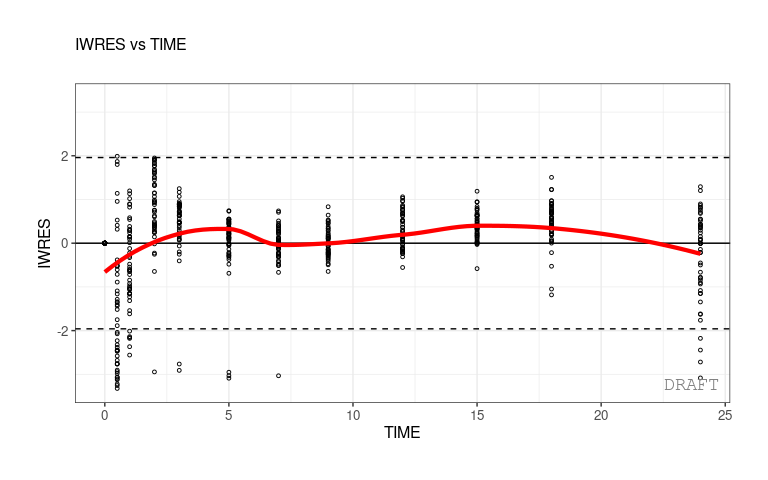# Diagnostics report

A report (in pdf and docx format) containing all default diagnostic plots can be created using the pmx_report function. The format can take three different values:

• ???report???: produces a pdf and a docx file (named `name.pdf` and `name.png` specified in argument name, located in save_dir) with default diagnostic plots
• ???plots???: produces a folder named `ggpmx_GOF` located in save_dir that contains all default diagnotic plots, each in a pdf and png file. The different plots are numerated in order to have an unique identifier for each plot (ex: ebe_box-1.pdf). This is necessary for having correct footnotes that indicated the path to the source file (for submission reports).
• ???both???: is a combination of both options above.

Example:

``````ctr %>% pmx_report(name='Diagnostic_plots2',
save_dir = work_dir,
format='both')``````

Note that running the same command first with the option ???format=???plots?????? and then with the option ???format=???report?????? will remove the ggpmx_GOF folder.

Note also that by default, the report will have the DRAFT label on all plots. The label can be removed by using the settings argument in the Controller creation, as described in Section 6.3.1.

The user can customize the default report by creating a ???template???. To create a template, the user should create first a default report with the following command:

``````ctr %>% pmx_report(name='Diagnostic_plots1',
save_dir = work_dir,
format='report')``````

The Rmarkdown (.Rmd) file is the ???template???. The user can modify the Rmarkdown file as desired (ex: changing the size of some figures) and save the modified file. The new template can be used with the following command:

``````ctr %>% pmx_report(name='Diagnostic_plots3',
save_dir = work_dir,
format='report',
template=file.path(work_dir,'Diagnostic_plots1.Rmd'))``````

# Customizing plots

Any particular plot can be customized in two ways:

• Specifying options in each call of a plot (on the fly, recommended):
``ctr %>% pmx_plot_xx(list of options)``
• Customizing a type of plot for all subsequent calls in the session by modifying the Controller:
``ctr %>% pmx_update(???xx???, list of options)``

Help(pmx_gpar) lists some options.

Help(pmx_plot_xx) lists some possible parameters to update a particular plot.

To obtain an exhaustive list of possible options for a particular plot, use the following:

``ctr %>% get_plot_config("xx")``

## BLQ

``````ctr = theophylline()
bloq = list(show = FALSE, color = "blue", alpha = 0.2, size = 3, pch = 8)``````

## Customizing global settings - `pmx_settings()`

The user can define a Controller with global settings that will be applied to all plots. For example remove draft annoataion, use abbreviation defintions to define axis labels, etc.

A settings object is defined by using the function `pmx_settings()`. The created object is passed as the parameter ???settings??? to `pmx()`. By doing so, the settings are defined globally and apply to all plots. For a complete list of global settings with their corresponding default values, please consult the ggPMX Help (`?pmx_settings`).

``````
## set one or more settings
my_settings <- pmx_settings(
is.draft   = FALSE,
use.abbrev = TRUE,
...) ### set other settings parameters here
ctr <-
pmx_mlx(
..., ## put here other pmx parametes
settings = my_settings
) ``````

### Remove DRAFT label globally

By default in the ???standing??? configuration, a DRAFT label is printed on all plots. In order to switch this label off, the user sets the `is.draft` option of `pmx_settings()` to `FALSE`.

``````
ctr <- theophylline(settings = pmx_settings(is.draft = FALSE))``````

### Use abbreviation definitions

The standing configuration initializes all plots using abbreviations for axis labels. Each abbreviation has its corresponding definition. To get the list of abbreviation :

``````ctr %>% get_abbrev
#> AIC : Akaike information criterion
#> BIC : Bayesian information criterion
#> BLQ : Below the limit of quantification
#> COAR : Clinical Operations Analytics and Regions
#> DV : Dependent variable
#> ETA : Inter-individual random effect
#> EBE : Empirical Bayes estimate
#> FO : First order
#> FOCE : First order conditional estimation
#> FOCEI : First order conditional estimation with interaction
#> IIV : Inter-individual variability
#> IPRED : Individual prediction
#> LRT : Likelihood ratio test
#> M&S : Modeling & Simulation
#> NLME : Nonlinear mixed-effects (model)
#> NPD : Normalized prediction discrepancy
#> NPDE : Normalized prediction distribution error
#> OCP : Oncology Clinical Pharmacology
#> OFV : Objective function value
#> PD : Pharmacodynamics
#> PK : Pharmacokinetics
#> PDF : Probability density function
#> SAEM : Stochastic approximation of the expectation-minimization algorithm
#> VPC : Visual predictive check
#> PRED : Population parameters predictions
#> EPRED : Population averaged predictions
#> IWRES : Individual weighted residuals
#> |IWRES| : |Individual weighted residuals|
#> NVS : Novartis internal report
#> HA : Report submitted to Health Authorities
#> TIME : Time after first dose (hours)``````

You can update one abbreviation to set a custom label

``ctr %>% set_abbrev(TIME="TIME after the first dose")``

Using `use.abbrev` flag you can use abbreviation definition to set axis labels:

``````ctr <- theophylline(settings=pmx_settings(use.abbrev = TRUE))
ctr %>% set_abbrev(TIME="Custom TIME axis")
ctr %>% pmx_plot_npde_time``````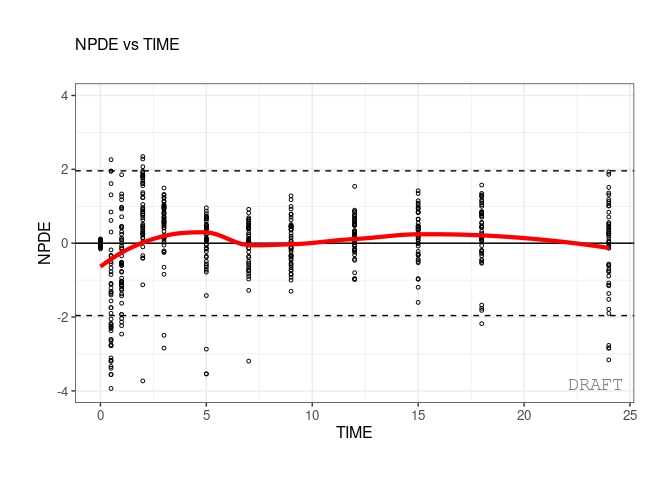### Use `finegrid.txt` file for individual plots

within Monolix, user can choose to not use finegrid file even if it is present.

``````ctr <- theophylline()
ctr %>% pmx_plot_individual(use.finegrid =FALSE, legend.position="top")
#> USE predictions data set``````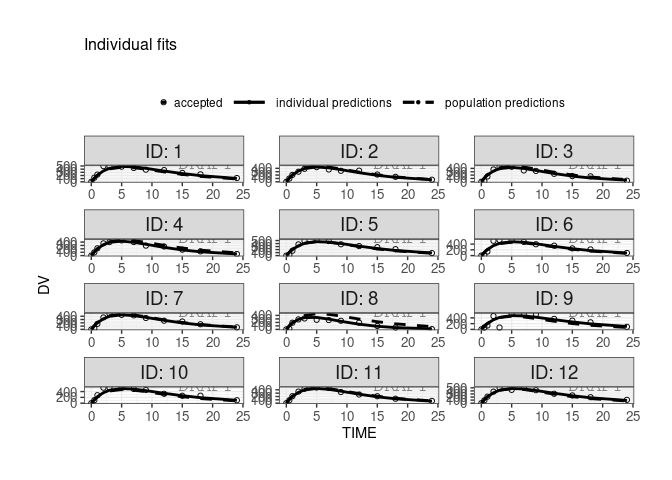### Set stratification color legend

In case of color startfication user can customize the legend. For example here using the `ggplot2::scale_color_manual`:

``````ctr <- theophylline()
ctr %>% pmx_plot_npde_time(strat.color="STUD")+
ggplot2::scale_color_manual(
"Study",
labels=c("Study 1","Study 2"),
values=c("1"="green","2"="blue"))``````Another way to do it is to define a global `scales.color` parameter that will applied to all plots with strat.color :

``````
ctr <- theophylline(
settings=
pmx_settings(
color.scales=list(
"Study",
labels=c("Study 1","Study 2"),
values=c("1"="orange","2"="magenta"))
)
)

ctr %>% pmx_plot_npde_time(strat.color="STUD")``````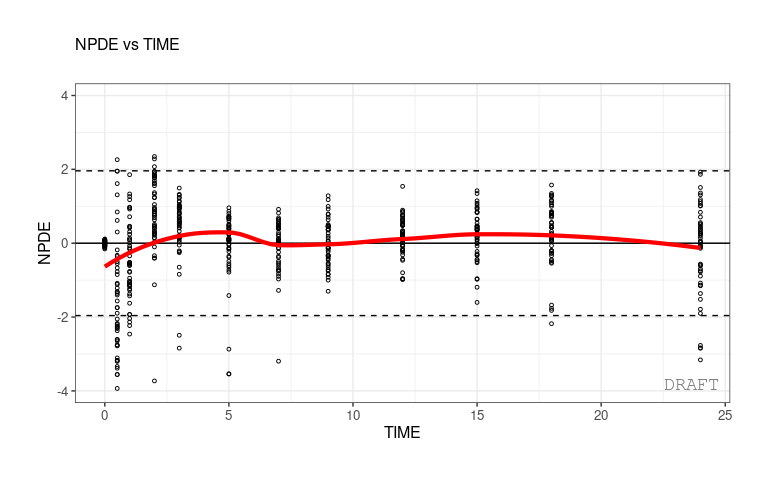``ctr %>% pmx_plot_eta_box(strat.color="STUD")``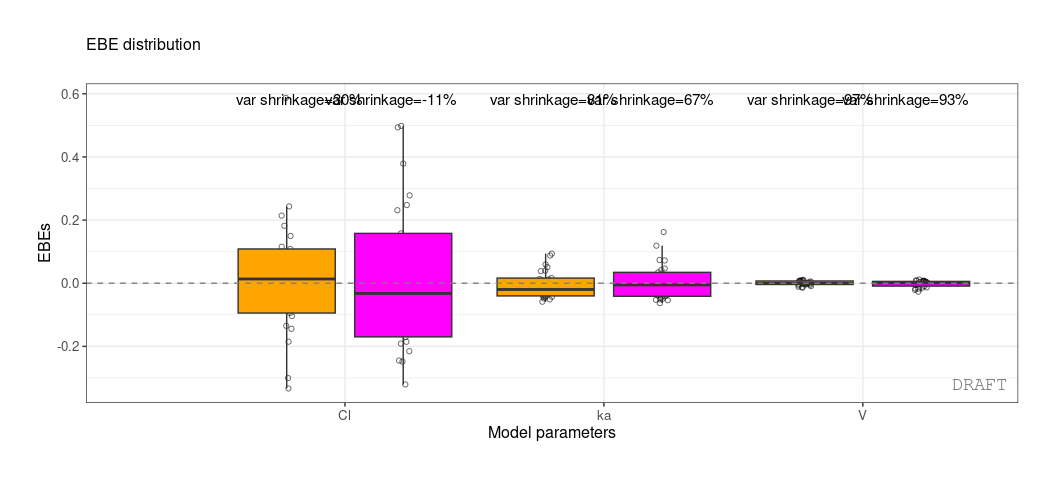### Define labels of categorical variables

In case of faceting by stratification user can redfine categorical labels to have more human readables strips. Lables are defined within `cats.labels` argument and user can use them by setting `use.lables` to TRUE.

``````

ctr <- theophylline(
settings=
pmx_settings(
cats.labels=list(
SEX=c("0"="M","1"="F"),
STUD=c("1"="Study 1","2"="Study 2")
),
use.labels = TRUE
)
)

ctr %>% pmx_plot_npde_time(strat.facet=~SEX)``````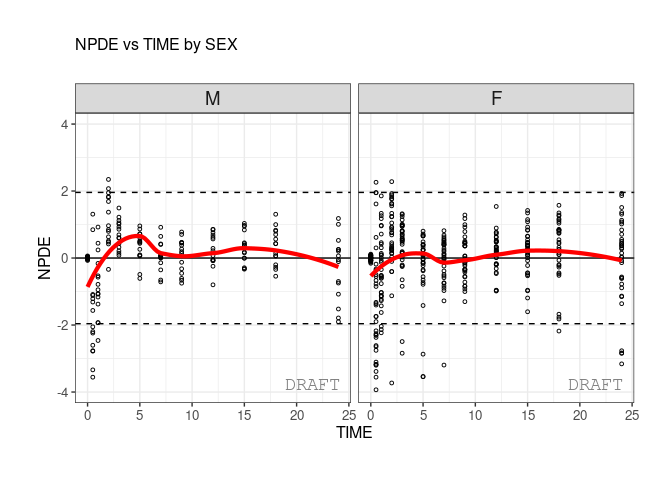``````

ctr <- theophylline(
settings=
pmx_settings(
cats.labels=list(
SEX=c("0"="M","1"="F"),
STUD=c("1"="Study 1","2"="Study 2")
),
use.labels = TRUE
)
)

ctr %>% pmx_plot_npde_time(strat.facet=~SEX)````````ctr %>% pmx_plot_eta_box(strat.facet =~SEX)``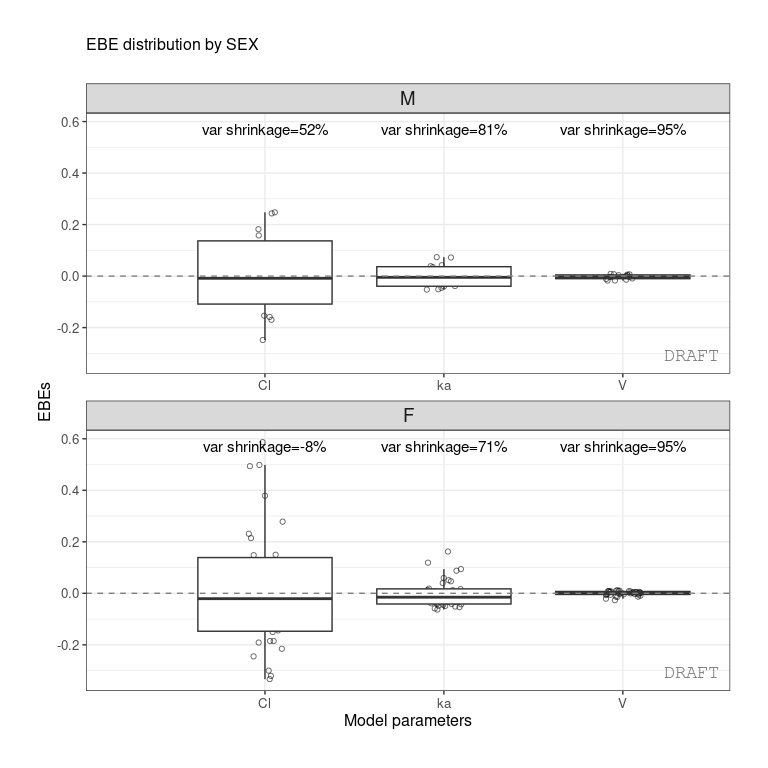# Appendix A

## Monolix requirements

ggPMX is compatible with Monolix versions 2016 and later. In order to be able to produce all available diagnostic plots, the following tasks should be executed in Monolix during the model fitting procedure:

• Population parameters;
• Individual parameters (EBEs);
• Standard errors;
• Plots.

In addition, make sure to export charts data (In Monolix 2018: Settings -> Preferences -> Export -> switch on the Export charts data button). Select at least the following plots to be displayed and saved: individual fits and scatter plot of the residuals.

## Plots table

The main target of ggPMX is to create a report containing the following plots (see abbreviation list below):

Abbreviations:

• NPDE: normalized prediction distribution errors
• IWRES: individual weighted residuals
• EBE: empirical Bayes estimates
• RE: random effects
• VPC: visual predivtive check

## ggPMX main functions

`ggPMX` implements few functions to generate and manipulate diagnostic plots. (Should we list pmx and pmx_mlx separately and say the differences? Or it???s maybe clear from the previous sections.)

(Apparently, it???s not the full list. Add all functions.) The design of the package is around the central object: the controller. It can introspected or piped using the `%>%` operand.

Note that:

The controller is an `R6` object, it behaves like a reference object. Some functions (methods) can have a side effect on the controller and modify it internally. Technically speaking we talk about chaining not piping here. However, using `pmx_copy` user can work on a copy of the controller.

## ggPMX graphical parameters

Graphical parameters in `ggPMX` are set internally using the `pmx_gpar` function. A number of graphical parameters can be set for the different plot types.

``````args(pmx_gpar)
#> function (labels, axis.title, axis.text, ranges, is.smooth, smooth,
#>     is.band, band, is.draft, draft, discrete, is.identity_line,
#>     identity_line, scale_x_log10, scale_y_log10, color.scales,
#>     is.legend, legend.position)
#> NULL``````

More information can be found in the help document `?pmx_gpar` and in the examples that follow.

## Pre-defined configurations

For the moment we are mainly using standing configuration. In the next release user can specfiy configuration either by cretaing a custom yaml file or an R configuration object. Also ggPMX will create many helper functions to manipulate the configuration objects.

## Default call

The shrinkage is a computation within controller data. In general it is used to annotate the plots. Although one can get it independently from any plot using `pmx_comp_shrink`. It is part of the `pmx_compt_xx` layer( In the future we will add , `pmx_comp_cor` , `pmx_comp_summary`,..)

Here the basic call:

``````ctr %>% pmx_comp_shrink
#>    EFFECT   OMEGA    SHRINK       POS FUN
#> 1:     Cl 0.22485 0.1125175 0.2934250 var
#> 2:      V 0.03939 0.9469996 0.0057915 var
#> 3:     ka 0.10024 0.7423478 0.0810850 var``````

We get the shrinkage for each effect (SHRINK column).

The same values can be shown on distribution plot , for example :

``ctr %>% pmx_plot_eta_box``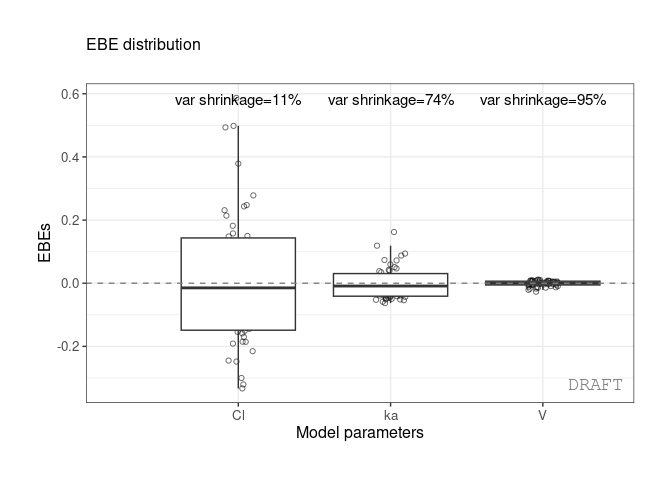or :

``ctr %>% pmx_plot_eta_hist``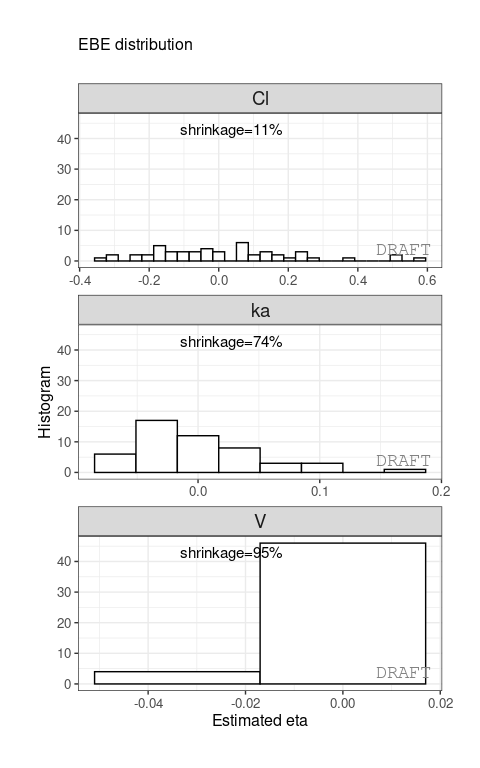You can add or remove shrinkage annotation using `is.shrink` argument ( TRUE by default) :

``ctr %>%   pmx_plot_eta_box( is.shrink = FALSE) ``## Var function

You can compute shrinkage by applying either standard deviation `sd` or variance `var` :

``````ctr %>% pmx_comp_shrink(  fun = "var")
#>    EFFECT   OMEGA    SHRINK       POS FUN
#> 1:     Cl 0.22485 0.1125175 0.2934250 var
#> 2:      V 0.03939 0.9469996 0.0057915 var
#> 3:     ka 0.10024 0.7423478 0.0810850 var``````
``ctr %>% pmx_plot_eta_box( shrink=list(fun = "var"))``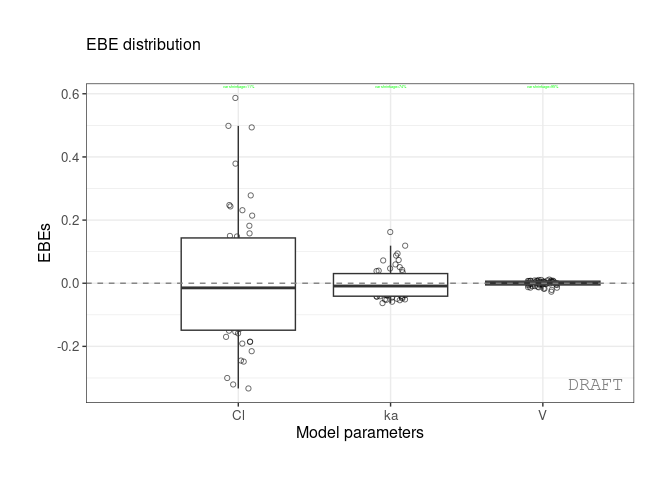## Shrinkage and stratification

Shrinkage can be applied after stratification :

``````ctr %>% pmx_comp_shrink(strat.facet = ~SEX)
#>    EFFECT SEX   OMEGA      SHRINK        POS FUN
#> 1:     Cl   1 0.22485 -0.08032359 0.29342500 var
#> 2:     Cl   0 0.22485  0.51828810 0.12378000 var
#> 3:      V   1 0.03939  0.94628054 0.00579150 var
#> 4:      V   0 0.03939  0.94818243 0.00437235 var
#> 5:     ka   1 0.10024  0.70737008 0.08108500 var
#> 6:     ka   0 0.10024  0.80907530 0.03676950 var``````

or by grouping like :

``````ctr %>% pmx_comp_shrink(strat.color = "SEX")
#>    EFFECT SEX   OMEGA      SHRINK        POS FUN
#> 1:     Cl   1 0.22485 -0.08032359 0.29342500 var
#> 2:     Cl   0 0.22485  0.51828810 0.12378000 var
#> 3:      V   1 0.03939  0.94628054 0.00579150 var
#> 4:      V   0 0.03939  0.94818243 0.00437235 var
#> 5:     ka   1 0.10024  0.70737008 0.08108500 var
#> 6:     ka   0 0.10024  0.80907530 0.03676950 var``````

We can

``````ctr %>% pmx_plot_eta_hist(is.shrink = TRUE, strat.facet = ~SEX,
facets=list(scales="free_y"))``````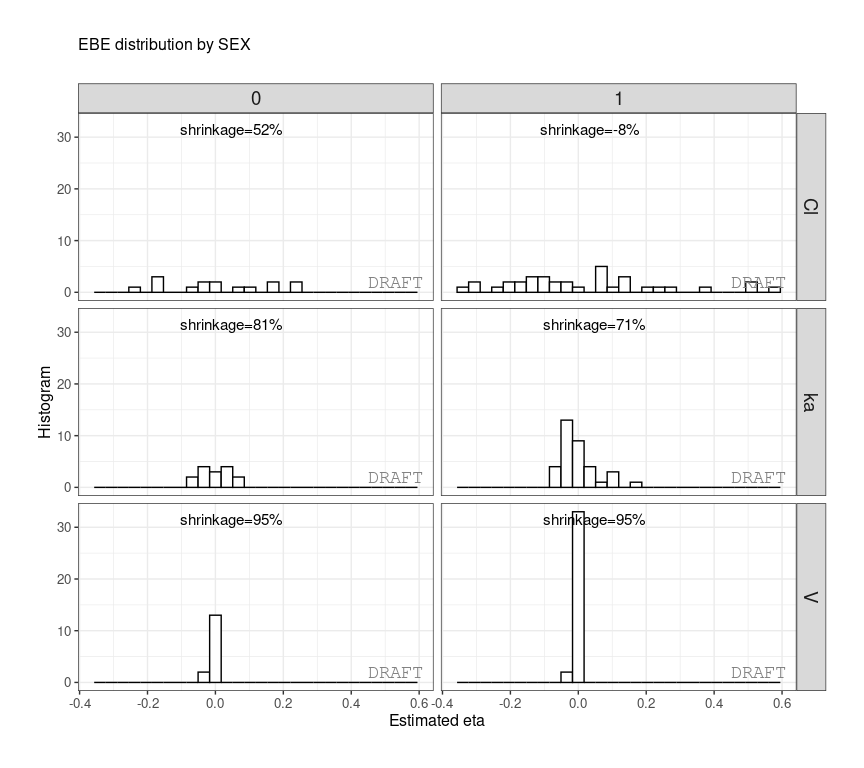or

``````ctr %>% pmx_plot_eta_box(is.shrink = TRUE, strat.facet = "SEX",
facets=list(scales="free_y",ncol=2))``````